Tento web obsahuje aplikace Google Adsense a Google analytics, které využívají data ze souborů cookie, více informací. Používání této stránky vyjadřujete souhlas s využitím těchto dat. Využívání dat ze souborů cokie lze zakázat v nastavení Vašeho prohlížeče.

# 40. FLOW OF GASES AND STEAM THROUGH NOZZLES

Jiří Škorpík, skorpik.jiri@email.cz
 Introduction . . . . . . . . . . . . . . . . . . . . . . . . . . 1 Converging nozzle . . . . . . . . . . . . . . . . . . . . . 1 Ideal contour of converging nozzle . . . . . . . . . . . . 3 State at exit of converging nozzle . . . . . . . . . . . . 4 Frequent contours of de Laval nozzles . . . . . . . . . 6 Flow through oblique cut nozzle . . . . . . . . . . 10 Flow through nozzle at losses . . . . . . . . . . . . 11 Efficiency of nozzle . . . . . . . . . . . . . . . . . . . . . 12 Some applications of nozzle theory . . . . . . . . 13 Nozzle as blade passage . . . . . . . . . . . . . . . . . 13 Rocket engine . . . . . . . . . . . . . . . . . . . . . . . . . 14 Flow through group of nozzles, flow through group of turbine stages . . . . . . . . . . . . . . . . . . . . . . . . 15 References . . . . . . . . . . . . . . . . . . . . . . . . . . . 16 Appendices . . (13 pages – only in Czech language)
 Full PDF version of the article with the appendices be can buy for 3.20 USD. More info here. Buy full text of article
The article of online continued resource Transformační technologie; ISSN 1804-8293;
www.transformacni-technologie.cz; Copyright©Jiří Škorpík, 2006-2019. All rights reserved. This work was published without any linguistic and editorial revisions.

## Introduction

A nozzle is a channel with stepless variable of flow area. Flowing of fluid through the nozzle is a process especially with a decrease pressure and an increase kinetic energy.

## Converging nozzle

The velocity of gas at the exit of the nozzle is function pressure at the inlet pi and at the exit pe (back-pressure) of the nozzle:1.416 State change of gas inside the nozzle.
A [m2] area of flow; c [m·s-1] velocity of gas; i [J·kg-1] specific enthalpy of gas; s [J·kg-1·K-1] specific entropy; t [°C] temperature of gas; p [Pa] pressure of gas. The subscript i denotes the state at the inlet of nozzle, the subscript e denotes the state at the exit of nozzle and the subscript c denotes the stagnation state of the gas.

Equation of outlet velocity can be derived from First law of thermodynamics for open system:2.101 The flow velocity at the exit of the nozzle in i-s diagram at isentropic expansion(1).
left formulas for calculation of outlet velocity of gas as function of static state of gas at inlet of nozzle; right formulas for calculation of outlet velocity of gas as function of stagnation state of gas at inlet of nozzle. κ [-] heat capacity ratio; r [J·kg-1·K-1] individual gas constant; T [K] absolute temperature of gas; p [Pa] pressure of gas; ε [-] pressure ratio of static pressures (pe/pi); εc [-] pressure ratio at stagnation inlet pressure (pe/pic). This equation is called Saint Vénantova-Wantzel equation [2, p. 350]. This equation is derived for flow of ideal gas without friction and at negligible change of potential energy. The derivation of the equation for a calculation of the velocity of gas at the exit of the nozzle is shown in the Appendix 101.
(1)Remark
For description of liquid flow through nozzle (change density is negligible) is use Bernoulli equation.
● 1 ●
● 40. Flow of gases and steam through nozzles ●

The velocity of gas ce is function the inlet temperature Ti and pressure pi according the Equation 2 and a maximum velocity is reached at expansion in the vacuum pe=0:3.514 Velocity of gas at the exit of a nozzle.pat [Pa] atmospheric pressure. Gas properties: κ=1,4, r=287 J·kg-1·K-1, ti=20 °C, pi=pat, ci=0.

The mass flow rate of gas through the nozzle is calculated from the continuity equation:4.334 The equation of mass flow rate through the nozzle.
m [kg·s-1] mass flow rate of gas through nozzle; v [m3·kg-1] specific volume of gas; χm [-] variable parts of equation that is function of pressure ratio. The derivation of the equation for a calculation of the mass flow rate of gas through the nozzle is shown in the Appendix 334.

According this equation is true, if the pressure on the exit nozzle pe is decreasing then the mass flow rate m is increased only to a pressure ratio ε where the mass flow rate should be decreasing:5.515 The maximum of mass flow rate of gas through the nozzle.The curve 1-a-0 corresponds to Equation 4(2). The maximum mass flow rate m* is reached at a pressure ratio ε*c. According Equation 4 should following a mass flow rate fall. In really the mass flow rate is a constant and equals m* from point ε*c to an expansion to vacuum εc=0. The pressure ratio for the maximum mass flow rate of gas through the nozzle is called the critical pressure ratio (symbol of asterisk*). The derivation of the equation for a calculation of the critical pressure ratio of gas is shown in the Appendix 515.
(2)Bendemann ellipse
The curve 1-a-0 is similar with an ellipse, therefore this curve is usually substituted by the ellipse for case routine calculations. This ellipse is called Bendemann ellipse:
● 2 ●
● 40. Flow of gases and steam through nozzles ●6.162 Approximate calculation of mass flow rate through the nozzle according Bendemann ellipse.This formula can be use only for pe≥p*. The derivation of the equation of Bendemann ellipse is shown in the Appendix 162.

The heat capacity ratio κ can be various for individual types of gases and therefore their critical pressure ratios can be also various:

```gas  ε*c [-]   gas               ε*c [-]
------------   -------------------------
H    0,527     air (dry)         0,528
He   0,487     superheated steam 0,546
CO2  0,540     saturated steam   0,577  ```
7.699 The critical pressure ratios of some gases.

Gas velocity reaches the speed of sound at the critical and a lower pressure ratio in the nozzle throat (the narrowest area of the nozzle), this state of the flow is called a critical state of flow. The equations of flow for narrowest area of the nozzle be can derived by substituting equations for the critical pressure ratio (Equation 5) to the above equations for the gas velocity and mass flow rate:8.516 The equations of the critical flow in narrowest area of the nozzle.
These quantities are called critically (critical velocity, critical mass flow rate, critical pressure ratio...). χmax these constants are listed in a tables according type of gases and pressure ratio at ci=0; i* [J·kg-1] critical enthalpy (at critical enthalpy reaches isentropic expansion the critical velocity).

3D plot of the equation for mass flow rate of gas as function the inlet pressurea and the back-pressure is called flow rate cone of the nozzle.

The air flows through a nozzle, its velocity is 250 m·s-1, its pressure is 1 MPa, its temperature is 350 °C at the inlet of the nozzle. Surroundings pressure behind the nozzle is 0,25 MPa. The narrowest area of the nozzle has 15 cm2. (a) find if the flow through the nozzle is critical flow. (b) calculate the outlet velocity and (c) the mass flow rate of air. The properties of air are: cp=1,01 kJ·kg-1·K-1, r=287 J·kg-1·K-1, κ=1,4. You do not solve a situation behind the nozzle. The solution of this problem is shown in the Appendix 102.
Problem 1.102

## Ideal contour of converging nozzle

An ideal contour of the nozzle is smooth, parallel with streamlines (on the inlet even the exit to avoid not a rise of turbulence through sudden change of direction of flow velocity at the wall), on the exit must be uniform velocity field (this condition is confirmed by experiments [4, p. 319]). It means the outlet velocity should be in axial direction of the nozzle. This condition must also satisfy the streamlines at the wall of the nozzle:

● 3 ●
● 40. Flow of gases and steam through nozzles ●9.475 Influence contour of the nozzle on the direction of the outlet velocity.
(a) cone nozzle; (b) ideal contour of nozzle; (c), (d), (e) usually contours of nozzles; (c) so called Vitoshinsk nozzle or Vitoshinsk converging nozzle [4, p. 320], [16, p. 13] (use for reduction passage between two passages with different diameter or as blower nozzle of wind tunels); (d) contour of nozzle by lemniscate ; (e) contour of nozzle for outlet of bottles (rr≐1,5·re [5, p. 80]); r [m] radius of nozzle; l [m] length of nozzle. The cone nozzle has lower a mass flow coefficient than ideal contour nozzle (see Formula 23).

These types of contours be can use for non-circular passages and blade passages.

## State at exit of converging nozzle

The free flow at the exit of the nozzle can have two states(3, 4):

(3)The velocity at the exit corresponds sub-critical or critical pressure ratio, pe≥p*.
The outlet gas stream is deformed and gradually mixes with the surrounding gas. In this case the state of the outlet gas (velocity, temperature, pressure) will be the same as the surrounding gas at some distance from the exit of the nozzle – the outlet gas is in thermodynamic balance with the surrounding gas: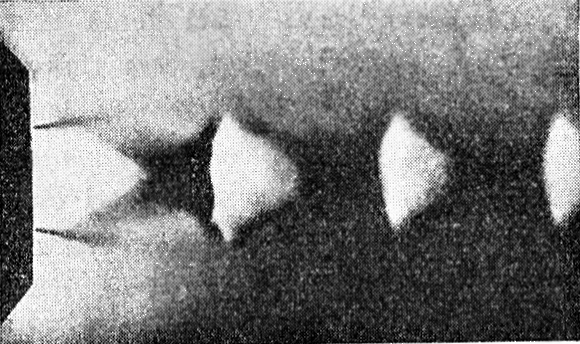10.984 The outflow from a converging nozzle at the critical pressure ratio.Photo from [3, p. 5].
● 4 ●
● 40. Flow of gases and steam through nozzles ●
(4)The pressure ratio is less than the critical ratio, pe<p*.
Expansion behind the nozzle continues and the velocity of gas increases to supersonic according the Equation 2. The gas stream area be must increased according Hugoniot condition. The divergent gas stream forms oblique shock waves on border between the stream and the surroundings gas. These shock waves are reflected to the core of gas stream and they are decreased an efficiency of expansion (they cause pressure drop). The expansion is ended when the pressure is equal the surroundings pressure and a next process is similar the previous case (gradually mixes with the surrounding gas).

## De Laval nozzle (converging-diverging nozzle)

For better efficiency of gas expansion behind the narrowest area of the converging nozzle (it is the case p*>pe) is necessary made the appropriate conditions. It means a divergent channel must be added to the converging nozzle behind narrowest flow area of the nozzle (so called critical flow area, because the speed of sound is reached here) – this design is called as De Laval nozzle:11.103 De Laval nozzle (CD nozzle)-direction of expansion.(a) converging section of nozzle; (b) divergent section of nozzle. Ma [-] Mach number; l [m] lengh of diverging section of nozzle. The velocity of gas is subsonic Ma<1 in the converging section, and is sonic Ma=1 in the narrowest area (throat), is supersonic Ma>1 in the diverging section.12.983 Supersonic exhaust of gas from de Laval nozzle.The oblique lines inside flow are oblique shock waves, which arise at the exit edge of the nozzle and are reflecting from the boundary of the stream. Photo from [3, p. 23].
● 5 ●
● 40. Flow of gases and steam through nozzles ●13.517 i-s diagram used in the description ideal expansion of gas through a CD nozzle.

## Frequent contours of de Laval nozzles

Ideal contour of the diverging section of de Laval nozzle is designed by the method of characteristics(5). There are analytical methods of design of contour diverging nozzle, where the contour of the nozzle is approximated by a polynomial (first-order, second-order and the like)(6, 7).

(5)Ideal contour of de Laval nozzle
This type of the nozzle have the most uniform velocity field at the exit. The contour of the nozzle on interval t-e is calculated by the method of characteristics through construction expansion waves inside the nozzle. As boundary condition is used the initial radius rr at αe=0° (condition of the exit velocity) and the flow area at exit Ae [4, p. 341], [5, p. 79]. The length of the ideal contour of the nozzle is longer than the nozzle with linear contour, therefore has lower internal efficiency due to internal friction of the working gas. Ideal contour of de Laval nozzle is used in supersonic wind tunels, where is requirement significant uniform velocity field at the outlet: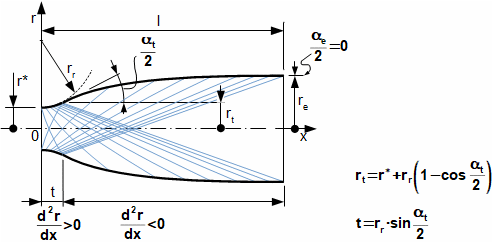14.993 Ideal contours of diverging section of de Laval nozzle.
α [°] angle of diverging section; t [m] inlet length of diverging section of de Laval nozzle (usually circle contour with radius rr≐0,382·r* [5, p. 80]). The ideal contour of nozzle is designed for maximum momentum of flow in axis direction. The expansion waves are shown on figure also. The derivation of equations for calculation the inlet length of diverging section of de Laval nozzle are shown in the Appendix 993.
● 6 ●
● 40. Flow of gases and steam through nozzles ●
(6)Linear (cone) contour of de Laval nozzle
These nozzles have simple calculation and simple manufactored, becouse has constant the angle α for whole length part t-e. The de Laval nozzles with cone contour are used as a supersonic blade row of one stage turbine (for cases where other losses of stage are very high and therefore production of complicated contour of nozzle does not economic). This simple contour is also used for small rocket engines, for small nozzles, for nozzle of injectors and ejectors etc. The calculation is composed from the specified of the angle of diverging α (usually in interval 8 up 30°) and from calculated the flow area at exit Ae. These two parameters are sufficient to a calculation of the length of the diverging section of de Laval nozzle:15.88 The linear contour of the Laval nozzle.
(a) equation of contour of nozzle for interval t-e; (b) equation of length of nozzle; (c) boundary conditions for calculation of constants a1, a2. The field velocity at the exit is not uniform. The deviation of the velocity from the axis causes losses momentum of the exit flow in axis direction (at the angle α=20° probably 1 % [5, p. 78]). The derivation of equation of the length of linear (cone) de Laval nozzle are shown in the Appendix 88.
(7)Bell nozzle
is the most common contour of the diverging section of de Laval nozzles for wide types of applications especially for rocket engines. This contour is descripted by an equation Rao (according Rao, G.V.R., which derived on base experiments , ) or by an equation Allman-Hoffman (according Allman J. G. and Hoffman J. D., which it was derived through a simplification of the Rao equation ). The Bell nozzle is shorter than the linear nozzle but has higher internal efficiency and the axial momentum of flow:16.335 The contour of Bell nozzle.
(a) equation of contour of nozzle for interval t-e by Rao; (b) equation of contour of nozzle for interval t-e by Allman-Hoffman; (c) boundary conditions for calculation of constants a1..a4 or b1..b3(8).
● 7 ●
● 40. Flow of gases and steam through nozzles ●
(8)Remark
For case of Rao equation are the boundary conditions for the inlet and the exit angle interdependent (αt=f(αe)). Choice of optimal pair of intial αt and the exit angle αe can be from length equivalent linear nozzle at α=30° see tables and charts in [5, p. 80]. The solving of Allman-Hoffman equation requires only the angle αt. This type of nozzle have approximately about 0,2% lower axial momentum of flow at expansion to vacuum than the nozzle profiled by Rao method . The Allman-Hoffman equation is used for quicker optimization calculations at wide combinations of the states at the exit.

A comparison all method of design of contour of the diverging nozzle are shown in .

Calculate a diverging section (cone contour) of the nozzle from the Problem 1. Calculate Mach number on the exit of the diverging section. The flare angle of the diverging section is 10°. The solution of this problem is shown in the Appendix 104.
Problem 2.104
Water steam flows through a de Laval nozzle. Pressure and temperature of the water steam is 80 bar respectively 500 °C at input to the nozzle. The leaving pressure is 10 bar. The mass flow rate of water steam must be 0,3 kg·s-1. Calculate base dimensions of the nozzle and state of water steam at the exit. The flare angle of the diverging section is α=10°. The solution of this problem is shown in the Appendix 336.
Problem 3.336

## Flow inside de Laval nozzle at non-nominal states

For case good design of de Laval nozzle is reached the pressure pn during expansion, which is the same as the back-pressure, this pressure is called the designated pressure of the nozzle. The non-nominalstate are changed the parameters of working gas at the inlet or the exit of nozzle. These parameters are changed from various causes (e.g. a control of the mass flow rate). If pe>pn (overexpansion nozzle) then the length of the divergent section of the nozzle is longer than it is need. If pe<pn (underexpansion nozzle) then the length of the divergent section of the nozzle is shorter than it is need. At pressure which is the bigger than the designated pressure can arise the normal shock wave inside de Laval nozzle.

A develop of the normal shock wave inside divergent section of the nozzle be can assumed from the Hugoniot condition. A smooth change the supersonic flow on the subsonic flow is allowed only in throat of the nozzle.

● 8 ●
● 40. Flow of gases and steam through nozzles ●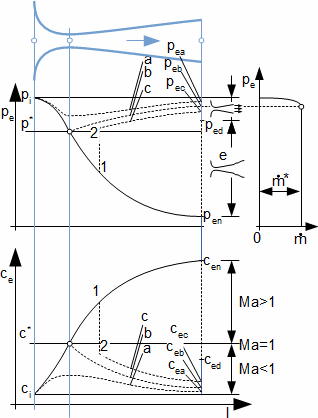17.105 De Laval nozzle – characteristics at several back-pressures(9-14).
Subscript 1 denotes a state in front the normal shock wave; index 2 denotes a state behind the normal shock wave.
(9)pe>pb
At this back-pressure the velocity at the throat does not reach speed of sound-it does not reach critical state, the curve a. Inside the converging section of the nozzle is done subsonic compression (increasing of pressure and decreasing of velocity-in this case the converging section of the nozzle works as a diffuser) to the pressure pa.
(10)pe=pb
At this back-pressure the velocity at the throat reaches speed of sound-critical state, the curve b. Inside the converging section of the nozzle is done subsonic compression to the pressure pb.
(11)pb>pe>pd
At this back-pressure the velocity at somewhere inside converging section of the nozzle occurs non-continuity of the velocity and pressure (is developed the normal shock wave). Behind the normal shock wave is subsonic flow and the gas is being compressed to pressure pc.
● 9 ●
● 40. Flow of gases and steam through nozzles ●
(12)pe=pd
At this back-pressure the normal shock waves arises exactly at the exit of the nozzle.
(13)pd>pe>pn
At this back-pressure the normal shock waves arises behind the exit of the nozzle. This normal shock wave is unsteady (free flow) and it alternately develops and disappears (similar situation is in case an converging nozzle where back-pressure is smaller than is the critical pressure pe<p*)
(14)pn>pe
At this back-pressure continued the expand of the working gas behind the exit of the nozzle. Similar as previous case arise sonic effects.

The position of normal shock wave inside the diverging section of the Laval nozzle is can calculate through the Rankine-Hugoniot equations for stable normal shock wave:

Find the approximate position of normal shock wave inside de Laval nozzle from Problem 2, if the back-pressure is increased about 0,52 MPa. The solution of this problem is shown in the Appendix 862.
Problem 4.862

The normal shock wave inside the nozzle is not usually stable [4, p. 363] therefore it can cause a vibration of the nozzle and connected machines, and increases noise.

A operation back-pressure has an influence on length of the nozzle of a rocket engine. During flight of a rocket through atmosphere is changed surrounding pressure with the altitude. Therefore the nozzles of first stage are designed on atmospheric pressure (pressure near ground) and the next stages are designed on smaller pressure (according the ignition altitude). The engines of last stage are designed on expansion to vacuum .

## Flow through oblique cut nozzle

If there is supersonic flow inside a oblique cut nozzle then the stream of the gas is deviated through an expansion fan from the nozzle axis. This expansion fan is developed on shortly side of the nozzle. Similar situation arises for case a blade passage at the end blade passage (see a chapter lower Nozzle as blade passage):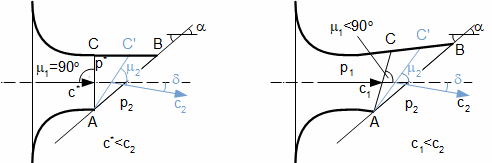18.106 Flow through an oblique cut nozzle-for a case the critical state in the throat.
left converging nozzle; right de Laval nozzle. μ [°] Mach angle; δ [°] deviation of stream from nozzle axis.
● 10 ●
● 40. Flow of gases and steam through nozzles ●

A change of the back-pressure p2 at the end of the converging nozzle influences the direct of the outlet stream through three possible ways(15-17):

(15)p2>p*
In the throat of the nozzle is the pressure p2 because the flow is subsonic. The direct of the outlet stream is the same as axis of the nozzle.
(16)p2=p*
If the back-pressure p2 is the critical pressure then this pressure must be in the throat of the nozzle. The velocity stream is equal the sound velocity at this location. The expansion is stopped and the stream does not deviate from the axis of the nozzle.
(17)p2<p*
The critical pressure is in the throat (cross section A-C) and the back-pressure is at the cross section A-C'. Between the cross section A-C and A-C' there is an expansion fan. The stream is deviated from the axis of nozzle with angle δ through the expansion fan.

The flow through oblique cut Laval nozzle is the same as the supersonic flow around an obtuse angle. The start of expansion is at pressure p1 on the cross section A-C and the end of expansion is at back-pressure p2 on the cross section A-C'. Therefore the oblique cut Laval nozzle is better for variable backpressure than classic Laval nozzle.

## Flow through nozzle at losses

In the previous paragraphs is described isentropic expansion of the working gas in the nozzle. But the expansion in the nozzle is influenced by a friction or also internal loss heat that arises through inner friction of the gas and a friction of gas on a wall of the nozzle. This friction heat decreases of the kinetic energy of the gas at the end of the nozzle. The friction heat is a loss between the kinetic energy at the nozzle exit for case isentropic expansion and the actual kinetic energy at the nozzle exit:19.108 Flow through the nozzle at the losses.z [J·kg-1] specific loss in the nozzle. Index iz denotes the state for case the isentropic expansion.
● 11 ●
● 40. Flow of gases and steam through nozzles ●

At pressure p*iz can be the velocity in core of the stream equal as sound velocity. But the velocity of flow can be subsonic on the periphery of the flow area due to boundary layer at the wall of the nozzle. Therefore the mean velocity at the throat of the nozzle is smaller than sound velocity respective the mean kinetic energy of the stream is smaller than kinetic energy for case sound velocity(18). The mean velocity stream is equal the sound velocity at the pressure p* see chapter viz kapitola 38. Flow of gas through channel with constant flow area.

(18)Remark
If the heat capacity ratio κ is not the same as at isentropic expansion, then konetic energy of speed sound is not the same as isentropic expansion (see speed of sound formula). It means, enthalpy is changed i*≠i*iz.

## Efficiency of nozzle

The nozzle loss is can calculated through energy parameters of the nozzle as a velocity coefficient φ and a nozzle efficiency η:20.569 The energy parameters of the nozzle.
φ [-] velocity coefficient; η [-] nozzle efficiency. The values of the velocity coefficients φ are shown in [4, p. 328] (for convergent nozzle including the cone nozzles) a [4, p. 348] (for Laval nozzles).

The description of the static state profile inside the nozzle or comparing two different nozzles cam be through a polytropic index. Mean value of the polytropic index can be derived from equation for difference of specific enthalpy between two states of the gas:21.883 The equation for calculation mean value of polytropic index between two state of gas.n [-] polytropic index.
Calculate the throat area and the exit area of the de Laval nozzle and its efficiency. Through the de Laval nozzle flows the water steam saturation. The mass flow rate is 0,2 kg·s-1. The stagnation pressure at the inlet of the nozzle is 200 kPa and back-pressure is 20 kPa. The velocity coefficient of the nozzle is 0,95. The solution of this problem is shown in the Appendix 109.
Problem 5.109

## Contraction of flow and mass flow coefficient

The mass flow is increased not only under internal friction of fluid but also under a contraction of flow behind narrowest area of the nozzle [15 p. 14]. The contraction of flow is caused by inertia flow and it has the same impact as an decreasing flow area of the nozzle:

● 12 ●
● 40. Flow of gases and steam through nozzles ●22.761 Contraction of flow inside the nozzle.A'min [m2] flow area of nozzle at contraction. The contraction for cases perfectly made nozzles is very small (Amin≈A'min), it is the bigger for case orfice plates.

Real mass flow of the nozzle is calculated by mass flow coefficient (discharge coefficient), which takes into account influence of internal losses and the contraction of flow. The mass flow coefficient is ratio the real mass flow of the nozzle and isentropic mass flow without any contraction:23.478 Mass flow coefficient.μ [-] mass flow coefficient.; m•iz [kg·s-1] mass flow rate at flow without loss (isentropic expansion).

Values of the mass flow coefficients any types of the nozzles and the orffice plates are shown in , .

## Some applications of nozzle theory

The theory of the nozzles can be aplicated on various type of flow devices. Through theory of the nozzles be can descripted complicated flow system.

## Nozzle as blade passage

The blade passage can have same shape as convergent nozzle or de Laval nozzle. The blade passage with de Laval profile is used for case supersonic velocity of working gas at the exit (decreasing of enthalpy between the inlet and the exit is under critical enthalpy i*). This type of blade passage has properties as oblique cut CD nozzle: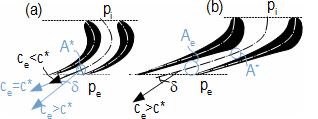24.111 The supersconic flow at the exit of blade passage.
(a) convergent blade passage; (b) blade passge for supersonic flow. A [m2] flow area; δ [°] deflection of supersonic flow from axis of passage. The procedure of calculation of angle δ is shown in [3, Equation 3.6-10] or be can use Prandtl-Meyer equation. Photos of high velocity flow of gas through blade rows are shown in chapter 16. Aerodynamics of airfoils and blade rows at compressible flow.
● 13 ●
● 40. Flow of gases and steam through nozzles ●

Blade passage with supersonic flow are occured usually in small one-stage turbine and last stages of condesing turbines.

## Rocket engine

Rocket engine is a reaction engine. The thrust of the engine is equal the momentum of exhaust gas flow at the exit. Main parts of the rocket engine is a combustion chamber and deLaval nozzle which is fastened at the exhaust of the combustion chamber. Inside the combustion chamber is burning an oxidizer and the fuel at develepment of the exhaust gas, which expands through the nozzle. Significant requirement is high velocity of the exhaust at the exit nozzle, because this is way reach of higher ration between the thrust and consumation of the fuel (ratio is called specific impulse). From a rearrange of the equation for the velocity at the exit of nozzle is evident, substances with high burning temperature and low molar weight, e.g. hydrogen (burning temperature of hydrogen is to TH2O=3517 K at molar mass MH2O=18 kg·mol-1) are the suitable fuels of the rocket engines:25.113 The liquid propellant rocket engine and formula of exhaust gas velocity at the exit.
1 oxidizer; 2 fuel; 3a pump oxidizer; 3b fuel pump; 4 combustion chamber; 5 exit of Laval nozzle; 6 resource of hot gasess for turbine; 7 turbine; 8 turbine exhaust. T [N] thrust; R [J·mol-1·K-1] Avogadro gas constant (8314 J·mol-1·K-1); M [kg·mol-1] molar mass of exhaust gas.

The solid propellant rocket engine use solid fuel. The hot exhaust gas is being arised at burning of the solid fuel. Thrust and burning of these engines not possible governing. Other side they are simpler than the liquid propellant rocket engines. There are hybrid solid propellant rocket engines with combination solid fuel and liquid oxidizer (this way be can regulation of thrust). The solid propellant rocket engines can be repeatedly use (e.g. the first stage of Space shuttle so called SRB):26.511 Solid propellant rocket engine.1 combustion chamber; 2 mix of fuel and oxidizer (its surface infuences mass flow rate of exhaust gas); 3 throat of nozzle; 4 diverging nozzle. Thrust vector is usually regulated through an oblique shock wave.
● 14 ●
● 40. Flow of gases and steam through nozzles ●

## Flow through group of nozzles, flow through group of turbine stages

The theory of the nozzles be can use for calaculation of a flow through a group of turbine stages at change of state of the gas in front of or behind this group of the stages. There are a few theories of calculation (e.g. v , ). These theories are not in use currently, because are used numerical method. I describe here only the simplest method. The method is usefull for aproximate calculation see also 25. Consumption characteristics of steam turbines at change state of steam.

The blade passages of one stage of the turbine can be compared with two nozzles which working at series(19). It means the mass flow rate through both nozzles is the same. The same assumption can be applied to the group of with a few stages or on group a few nozzles which are in row.

(19)The flow through the stage of the turbine as the flow through two nozzle in row
The flow through the blade passages of the rotor must be calculated from the relative velocity.

Sufficient solving of calculation of change flow mass rate through the group of the stages be can reached by use only two simplifying assumptions. The assumption of adiabatic expansion and its constant polytropic index at change of mass flow rate is the first assumption. The change of specific volume at flow of the working gas through one stage is negligible and specific volume is suddenly changed at the exit of stage, it is the second assumption: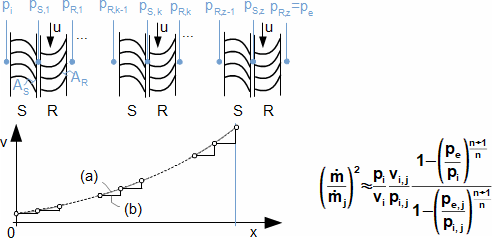27.994 Approximate formula of change of flow mass rate through the group of turbine stages.
(a) profile of specific volume inside stages; (b) profile of specific volume inside stage according second assumption. n [-] polytropic index of flow through group of stages; x [m] length of group of stages. Indexes: R rotor, S stator, j nominal state; z number of stages; k number of stage. Derivation of the equation of approximate calculation of change of flow rate through the group of turbine stages is shown in [14, p. 315].

Last equation be can simplification through Bendeman ellipse :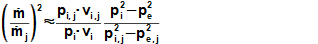28.995 Approximate formula of change of flow rate through the group of turbine stages which is derived use Bendeman ellipse.The derivation is shown in [13, p. 181].
● 15 ●
● 40. Flow of gases and steam through nozzles ●

The Equation 28 has a less accuracy than the Equation 27, but is simpler and its solve is the same as a quadratic equation. The Equation 27 has non-quadratic solve.

The Equations 27 and 28 are also accurate for saturation vapour, but the reading of specific volume is not sufficient at gas near his saturation.

If critical state is indicated at the last blade row of the group of stages, then be can use knowledge for critical flow through nozzle. It means that the equation for mass flow for these conditions must be same as the equation at expansion to vacuum (pe=0):29.996 Flow through the group of stages at critical pressure ratio of the last of blade row.Derived from Equation 28 for pe=0.

Last blade row with critical state are use e.g. by condensing turbine. An example of calculation change of flow rate through steam turbine is shown in chapter 25. Consumption characteristics of steam turbines at change state of steam.

## References

1. TOMEK, Petr. Kde jsou ty (skutečné) kosmické lodě?. VTM Science, 2009, leden. Praha: Mladá fronta a.s., ISSN 1214-4754.
2. KALČÍK, Josef, SÝKORA, Karel. Technická termomechanika, 1973. 1. vydání, Praha: Academia.
3. SLAVÍK, Josef. Modifikace Pitotova přístroje a jeho užití při proudění plynu hubicí, 1938. Praha: Elektrotechnický svaz Československý.
4. DEJČ, Michail. Technická dynamika plynů, 1967. Vydání první. Praha: SNTL.
5. SUTTON, George, BIBLARLZ, Oscar. Rocket propulsion elements, 2010. 8th ed. New Jersey: John Wiley& Sons, ISBN: 978-0-470-08024-5.
6. RAO, G. V. R. Exhaust nozzle contour for optimum thrust, Jet Propulsion, Vol. 28, Nb 6, pp. 377-382,1958.
7. ALLMAN, J. G. HOFFMAN, J. D. Design of maximum thrust nozzle contours by direct optimization methods, AIAA journal, Vol. 9, Nb 4, pp. 750-751, 1981.
8. W.B.A. van MEERBEECK, ZANDBERGEN, B.T.C. SOUVEREIN, L.J. A Procedure for Altitude Optimization of Parabolic Nozzle Contours Considering Thrust, Weight and Size, EUCASS 2013 5th European Conference for Aeronautics and Space Sciances, Munich, Germany, 1-5 July 2013.
9. HADDAD, A. Supersonic nozzle design of arbitrary cross-section, 1988. PhD Thesis. Cranfield institute of technology, School of Mechanical Engineering.
● 16 ●
● 40. Flow of gases and steam through nozzles ●
1. Autor neuveden. CONTOURING OF GAS-DYNAMIC CONTOUR OF THE CHAMBER . Web: http://www.ae.metu.edu.tr/seminar/2008/uyduitkilecture/doc5.pdf, [cit.-2015-08-24].
2. NOŽIČKA, Jiří. Osudy a proměny trysky Lavalovy, Bulletin asociace strojních inženýrů, 2000, č. 23. Praha: ASI, Technická 4, 166 07.
3. KADRNOŽKA, Jaroslav. Tepelné turbíny a turbokompresory I, 2004. 1. vydání. Brno: Akademické nakladatelství CERM, s.r.o., ISBN 80-7204-346-3.
4. KADRNOŽKA, Jaroslav. Parní turbíny a kondenzace, 1987. Vydání první. Brno: VUT v Brně.
5. AMBROŽ, Jaroslav, BÉM, Karel, BUDLOVSKÝ, Jaroslav, MÁLEK, Bohuslav, ZAJÍC, Vladimír. Parní turbíny II, konstrukce, regulace a provoz parních turbín, 1956. Vydání první. Praha: SNTL.
6. JARKOVSKÝ, Eduard. Základy praktického výpočtu clon, dýz a trubic Venturiho, 1958. Druhé vydání. Praha: Státní nakladatelství technické literatury.